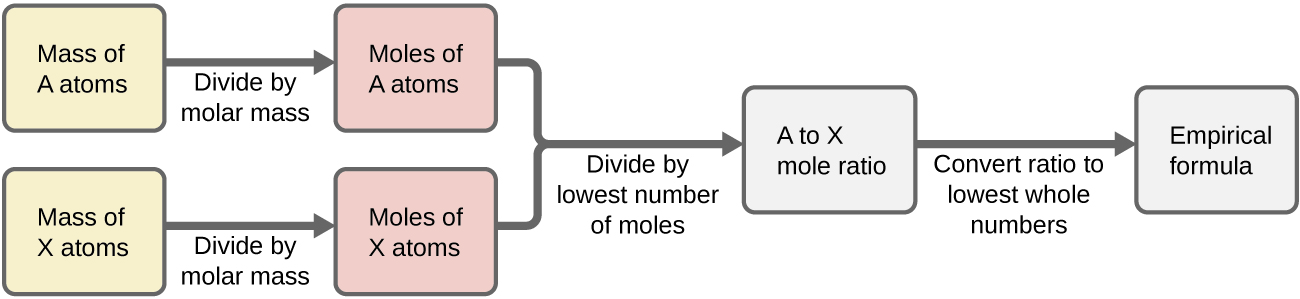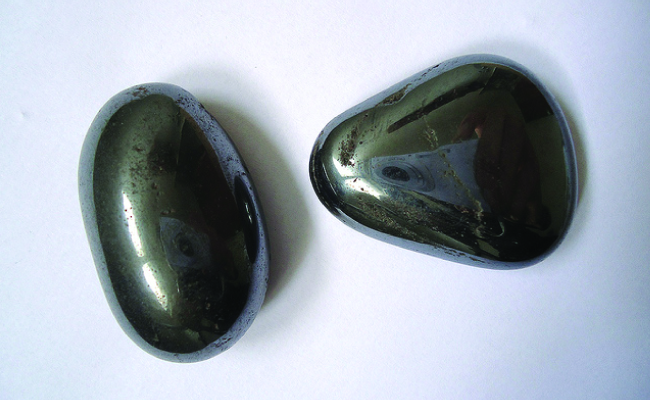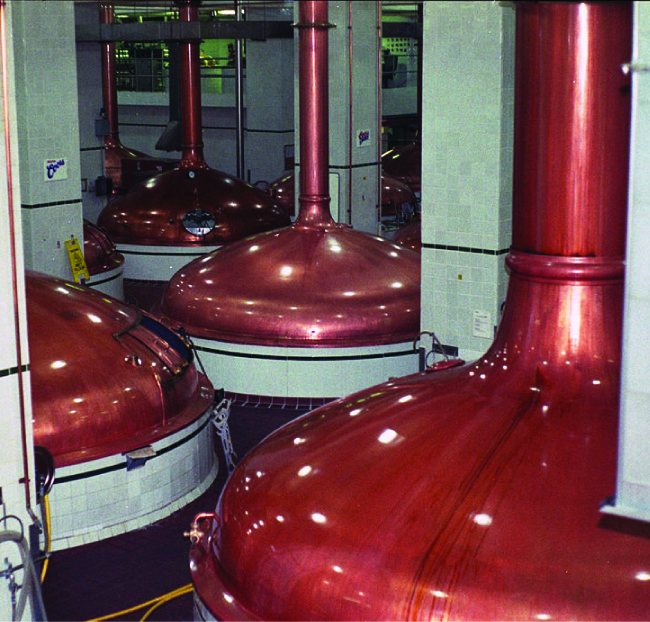# 3.2 Determining empirical and molecular formulas  (Page 3/6)

 Page 3 / 6
${\text{C1}}_{0.150}{\text{O}}_{0.525}={\text{Cl}}_{\phantom{\rule{0.2em}{0ex}}\frac{0.150}{0.150}\phantom{\rule{0.2em}{0ex}}}\phantom{\rule{0.2em}{0ex}}{\text{O}}_{\phantom{\rule{0.2em}{0ex}}\frac{0.525}{0.150}\phantom{\rule{0.2em}{0ex}}}={\text{ClO}}_{3.5}$

In this case, dividing by the smallest subscript still leaves us with a decimal subscript in the empirical formula. To convert this into a whole number, we must multiply each of the subscripts by two, retaining the same atom ratio and yielding Cl 2 O 7 as the final empirical formula.

In summary, empirical formulas are derived from experimentally measured element masses by:

1. Deriving the number of moles of each element from its mass
2. Dividing each element’s molar amount by the smallest molar amount to yield subscripts for a tentative empirical formula
3. Multiplying all coefficients by an integer, if necessary, to ensure that the smallest whole-number ratio of subscripts is obtained

[link] outlines this procedure in flow chart fashion for a substance containing elements A and X.The empirical formula of a compound can be derived from the masses of all elements in the sample.

## Determining a compound’s empirical formula from the masses of its elements

A sample of the black mineral hematite ( [link] ), an oxide of iron found in many iron ores, contains 34.97 g of iron and 15.03 g of oxygen. What is the empirical formula of hematite?Hematite is an iron oxide that is used in jewelry. (credit: Mauro Cateb)

## Solution

For this problem, we are given the mass in grams of each element. Begin by finding the moles of each:

$\begin{array}{l}\\ 34.97\phantom{\rule{0.2em}{0ex}}\text{g Fe}\left(\frac{\text{mol Fe}}{55.85\phantom{\rule{0.2em}{0ex}}\text{g}}\right)& =& 0.6261\phantom{\rule{0.2em}{0ex}}\text{mol Fe}\hfill \\ 15.03\phantom{\rule{0.2em}{0ex}}\text{g O}\left(\frac{\text{mol O}}{16.00\phantom{\rule{0.2em}{0ex}}\text{g}}\right)& =& 0.9394\phantom{\rule{0.2em}{0ex}}\text{mol O}\hfill \end{array}$

Next, derive the iron-to-oxygen molar ratio by dividing by the lesser number of moles:

$\begin{array}{}\\ \\ \phantom{\rule{0.2em}{0ex}}\frac{0.6261}{0.6261}\phantom{\rule{0.2em}{0ex}}=1.000\phantom{\rule{0.2em}{0ex}}\text{mol Fe}\\ \phantom{\rule{0.2em}{0ex}}\frac{0.9394}{0.6261}\phantom{\rule{0.2em}{0ex}}=1.500\phantom{\rule{0.2em}{0ex}}\text{mol O}\end{array}$

The ratio is 1.000 mol of iron to 1.500 mol of oxygen (Fe 1 O 1.5 ). Finally, multiply the ratio by two to get the smallest possible whole number subscripts while still maintaining the correct iron-to-oxygen ratio:

$2\left({\text{Fe}}_{1}{\text{O}}_{1.5}\right)\phantom{\rule{0.2em}{0ex}}=\phantom{\rule{0.2em}{0ex}}{\text{Fe}}_{2}{\text{O}}_{3}$

The empirical formula is Fe 2 O 3 .

## Check your learning

What is the empirical formula of a compound if a sample contains 0.130 g of nitrogen and 0.370 g of oxygen?

N 2 O 5

## Deriving empirical formulas from percent composition

Finally, with regard to deriving empirical formulas, consider instances in which a compound’s percent composition is available rather than the absolute masses of the compound’s constituent elements. In such cases, the percent composition can be used to calculate the masses of elements present in any convenient mass of compound; these masses can then be used to derive the empirical formula in the usual fashion.

## Determining an empirical formula from percent composition

The bacterial fermentation of grain to produce ethanol forms a gas with a percent composition of 27.29% C and 72.71% O ( [link] ). What is the empirical formula for this gas?An oxide of carbon is removed from these fermentation tanks through the large copper pipes at the top. (credit: “Dual Freq”/Wikimedia Commons)

## Solution

Since the scale for percentages is 100, it is most convenient to calculate the mass of elements present in a sample weighing 100 g. The calculation is “most convenient” because, per the definition for percent composition, the mass of a given element in grams is numerically equivalent to the element’s mass percentage. This numerical equivalence results from the definition of the “percentage” unit, whose name is derived from the Latin phrase per centum meaning “by the hundred.” Considering this definition, the mass percentages provided may be more conveniently expressed as fractions:

$\begin{array}{l}\\ 27.29%\phantom{\rule{0.2em}{0ex}}\text{C}& =\hfill & \phantom{\rule{0.2em}{0ex}}\frac{27.29\phantom{\rule{0.2em}{0ex}}\text{g C}}{100\phantom{\rule{0.2em}{0ex}}\text{g compound}}\phantom{\rule{0.2em}{0ex}}\hfill \\ 72.71%\phantom{\rule{0.2em}{0ex}}\text{O}& =\hfill & \phantom{\rule{0.2em}{0ex}}\frac{72.71\phantom{\rule{0.2em}{0ex}}\text{g O}}{100\phantom{\rule{0.2em}{0ex}}\text{g compound}}\phantom{\rule{0.2em}{0ex}}\hfill \end{array}$

The molar amounts of carbon and hydrogen in a 100-g sample are calculated by dividing each element’s mass by its molar mass:

$\begin{array}{l}\\ 27.29\phantom{\rule{0.2em}{0ex}}\text{g C}\left(\frac{\text{mol C}}{12.01\phantom{\rule{0.2em}{0ex}}\text{g}}\right)& =\hfill & 2.272\phantom{\rule{0.2em}{0ex}}\text{mol C}\hfill \\ 72.71\phantom{\rule{0.2em}{0ex}}\text{g O}\left(\frac{\text{mol O}}{16.00\phantom{\rule{0.2em}{0ex}}\text{g}}\right)& =\hfill & 4.544\phantom{\rule{0.2em}{0ex}}\text{mol O}\hfill \end{array}$

Coefficients for the tentative empirical formula are derived by dividing each molar amount by the lesser of the two:

$\begin{array}{}\\ \\ \phantom{\rule{0.2em}{0ex}}\frac{2.272\phantom{\rule{0.2em}{0ex}}\text{mol C}}{2.272}\phantom{\rule{0.2em}{0ex}}=1\\ \phantom{\rule{0.2em}{0ex}}\frac{4.544\phantom{\rule{0.2em}{0ex}}\text{mol O}}{2.272}\phantom{\rule{0.2em}{0ex}}=2\end{array}$

Since the resulting ratio is one carbon to two oxygen atoms, the empirical formula is CO 2 .

## Check your learning

What is the empirical formula of a compound containing 40.0% C, 6.71% H, and 53.28% O?

## Answer:

CH 2 O

#### Questions & Answers

what is molecule
Olom Reply
What is the generic name for the compound
Orisanmi Reply
what is the formular for methane
Tamaranimiweremi Reply
CH4 , it is the simplest alkane
Abdelkarim
what is the formula for alkaline
Olom
hi
Yusuf
I wish to learn to more of chemistry, can someone please teach me.
Yusuf
what is zero gravity
Blessing Reply
every object is that zero gravity
Rabiu
Probably when an object is in space and there are no nearby masses that pull her, and exert gravity
Abdelkarim
Alright. .good job
Rabiu
And all majesty to God, (وَهُوَ ٱلَّذِی خَلَقَ ٱلَّیۡلَ وَٱلنَّهَارَ وَٱلشَّمۡسَ وَٱلۡقَمَرَۖ كُلࣱّ فِی فَلَكࣲ یَسۡبَحُونَ) [سورة الأنبياء 33 And it is He who created the night and the day and the sun and the moon; all [heavenly bodies] in an orbit are swimming. General theory of relativity in Qur
Abdelkarim
what is molecule?
Olom
what is lattice energy
Getrude Reply
why is CO a neutral oxide and CO2 an acidic oxide
Emmanuel Reply
Because when CO2 dissolves in water forming a weak acid. CO does not dissolve in water as it has strong triple bond.
Abdelkarim
What is acid
Progress Reply
which donate H+ or accept lone pair of electron
Kajal
kinetic theory of matter and gas law
Victoria Reply
hi
Victoria
pls explain
Victoria
what is clay
Thankgod Reply
material containing clay minerals. Clays develop plasticity when wet, due to a molecular film of water surrounding the clay particles, but become hard, brittle and non–plastic upon drying or firing. Most pure clay minerals are white or light-coloured, but natural clays show a variety of colours
Abdelkarim
due iron oxide. The four types of clay are Earthenware clay, Stoneware clay, Ball clay, and Porcelain. All of them can be used to make pottery, but the end result would differ a lot thanks to their different textures, colors, and flexibilities.
Abdelkarim
And do you know that god has created human from clay (وَلَقَدۡ خَلَقۡنَا ٱلۡإِنسَـٰنَ مِن صَلۡصَـٰلࣲ مِّنۡ حَمَإࣲ مَّسۡنُونࣲ) [سورة الحجر 26] And We did certainly create man out of clay from an altered black mud. You can install Quran from paly store for free with translations.
Abdelkarim
darw a periodic table
Hazard Reply
draw a periodic table
Hazard
You will arrange the elements into row and coloumns according to increasing proton number. You may want to use symbols or their names. Hydrogen, Helium, etc. God has created all these elements from nothing, in Islam we know God is the creator.
Abdelkarim
why are you drawing a periodic table? why not just print one from the internet and use as a reference
Jakhari
Great thought
Bright
how are you?
Abel Reply
alright , how about you
Marina
am fine
Agbo
your name is Agbo?
Marina
my name is amel
Farid
l use the email of my husband
Farid
Define organic chemistry
Edward Reply
It is the chemistry concerning molecules that have Carbon skeletons and hydrogen atoms. We find organic molecules like in plants, living derivatives, etc.
Abdelkarim
what's matter
Joshua Reply
Anything that can be to cutting from all dimensions to halve. So you end up with 4 cubes of 5 cm side. Repeat with one of the cubes. 10, 5, 2.5, .., 0 1st 2nd 3rd Nth Un= a(r) ^ n-1
Abdelkarim
Anything that has mass and can reflect or absorb waves. GOD created everything from nothing only he can destroy it as prooved.
Abdelkarim
Suppose you have a cube of side 10 cm. Then you start cutting from all dimensions to halve. So you end up with 4 cubes of 5 cm side. Repeat with one of the cubes. 10, 5, 2.5, .., 0 1st 2nd 3rd Nth Un= a(r) ^ n-1 0= 10 (1/2)^n-1 0= (1/2) ^ n-1 Log0= (n-1) Log(1/2) - infinity =( n-1)
Abdelkarim
matter is anything that has mass,volume and can occupy space
Getrude
what is electrolysis
Rabi Reply
good equation
Aliyu
differenciate between fat and oil
Mustapha Reply

### Read also:

#### Get Jobilize Job Search Mobile App in your pocket Now!

Source:  OpenStax, Chemistry. OpenStax CNX. May 20, 2015 Download for free at http://legacy.cnx.org/content/col11760/1.9
Google Play and the Google Play logo are trademarks of Google Inc.

Notification Switch

Would you like to follow the 'Chemistry' conversation and receive update notifications?ByByBy OpenStaxBy OpenStaxBy Laurence BailenBy Dionne MahaffeyBy Anh DaoBy Madison ChristianBy Christine ZeelieBy Marion CabalfinBy Madison ChristianBy Kevin Amaratunga